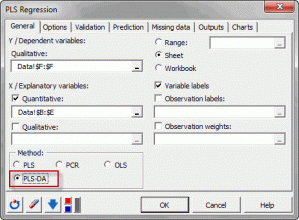PLS discriminant analysis has the same purposes as discriminant analysis but benefits from the advantages of PLS. In Excel using the XLSTAT software.## What is PLS discriminant analysis?

PLS regression can be adapted to fit discriminant analysis (PLS-DA). The PLS discriminant analysis uses the PLS algorithm to explain and predict the membership of observations to several classes using quantitative or qualitative explanatory variables.  XLSTAT uses the PLS2 algorithm applied on the full disjunctive table obtained from the qualitative dependent variable.

PLS discriminant analysis can be applied in many cases when classical discriminant analysis cannot be applied. For example, when the number of observations is low and when the number of explanatory variables is high. When there are missing values, PLS discriminant analysis can be applied on the data that is available. Finally, as PLS regression, it is adapted when multicollinearity between explanatory variables is high.

As many models as categories of the dependent variable are obtained. An observation is associated to the category that has an equation with the highest value.

PLS discriminant analysis offers an interesting alternative to classical linear discriminant analysis.

The output mixes the outputs of the PLS regression with classical discriminant analysis outputs such as confusion matrix.### analice sus datos con xlstat

prueba gratuita de 14 días

Incluido en